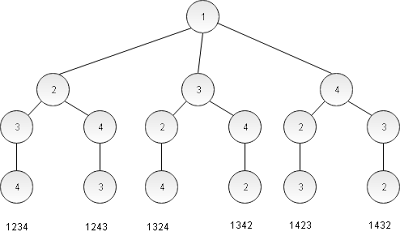## Thursday, November 23, 2017

### Generate all possible permutations in Java using recursion.

In the previous example, we drew a pyramid in java without any loop. We used recursion to achieve our goal and hopefully, you got a very clear idea how recursion works. In the following example, I will show you how to print all permutations of a given string. If the given string is "1234", then all possible permutations are:

1234
1243
1324
1342
1324
1342
1423
1432
.
.
.
4321

A total number of permutations are 24. ( 4! = 24 )

In this case, we are going to use our same weapon, recursion. One of the best ways to understand recursion is to draw a tree to illustrate the logic. For the above example, we can draw the tree in the following way -This is a partial tree, started only with 1. The complete tree has 3 more branches.

This is my solution -
``````
public class Permutation {

final private int MAXN = 7;
private int[] flag = new int[MAXN];
private String input = null;
StringBuffer tempString = null;

/**
* Recursively generates all permutations.
*
* @param index
* @param level
*/
private void generatePermutation(int index, int level) {

tempString.setCharAt(level, input.charAt(index));

flag[index] = 1;

for (int i = 0; i < input.length(); i++) {
if (flag[i] == 0) {
generatePermutation(i, level + 1);
}
}
if (level == input.length() - 1) {
System.out.println(tempString);
}

flag[index] = 0;

}

/**
* Read Input from Standard Input.
*/
private void readInput() {

Scanner scanner = new Scanner(System.in);
this.input = scanner.nextLine();

if (this.input.length() > 7) {
System.exit(0);
}
this.tempString = new StringBuffer(this.input);
for (int i = 0; i < this.input.length(); i++) {
this.generatePermutation(i, 0);
}
scanner.close();
}

public static void main(String[] args) {
}
}

``````
This is a pretty easy solution if you master in backtracking.

Well! can you modify the above program to generate unique permutations only? For an example, if you are given 112, then output should be-

112
121
211

Not like the above code produces:

112
121
112
121
211
211

As you see, there are some duplicate items are produced, which need to be removed. I am sure, you can do it.